### Day 46 - Completing the Square - 03.18.15

• Unit 4 Test on 3/27!
• If you are leaving for spring break early, please make arrangements with me to take the test before break!

Questions

Bell Ringer
1. What value of c makes this expression a perfect square: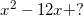1. -36

2. 36

3. 6

4. 24

5. none of the above

2. Convert the following to vertex form using completing the square: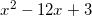1.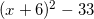2.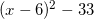3.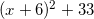4.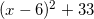5. none of the above

3. Convert the following to vertex form using completing the square: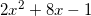1.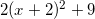2.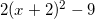3.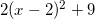4.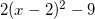5. none of the above

Review
• How are key features of a graph of a quadratic function found?
• Recognize that a quadratic function has the shape of a parabola.
• Find the coordinates of the vertex of a parabola.
• Recognize whether the vertex of a parabola represents a minimum or a maximum.
• Find the equation of the axis of symmetry.
• Find the x and y-intercepts of a quadratic function.
• How can quadratic functions be represented to best describe the desired key features?
• Recognize standard form of a quadratic function.
• Recognize factored form of a quadratic function.
• Recognize vertex form of a quadratic function.
• Determine which forms of a quadratic function are best used for solve a problem.

Lesson
• Completing the Square (video 1) (video 2)
• How can quadratic equations be solved using completing the square?
• Recognize perfect square quadratic equations.
• Create perfect square quadratic equations.
• Solve quadratic equations using completing the square.
• Activity
• Extra Practice 1
• Extra Practice 2

Exit Ticket
• Posted on the board at the end of the block
Lesson Objective(s)
• How can quadratic equations be solved using completing the square?
Skills
1. Recognize perfect square quadratic equations.
2. Create perfect square quadratic equations.
3. Solve quadratic equations using completing the square.

#### In-Class Help Requests

Standard(s)
• CC.9-12.F.IF.7a Graph linear and quadratic functions and show intercepts, maxima, and minima.
• CC.9-12.A.REI.4 Solve equations and inequalities in one variable. Solve quadratic equations in one variable.
• CC.9-12.A.REI.4b Solve quadratic equations by inspection (e.g., for x^2 = 49), taking square roots, completing the square, the quadratic formula and factoring, as appropriate to the initial form of the equation. Recognize when the quadratic formula gives complex solutions and write them as a ± bi for real numbers a and b.
• Factored Form
• CC.9-12.A.SSE.3a Factor a quadratic expression to reveal the zeros of the function it defines.
• CC.9-12.F.IF.8a Use the process of factoring and completing the square in a quadratic function to show zeros, extreme values, and symmetry of the graph, and interpret these in terms of a context.
• Completing the Square
• CC.9-12.A.SSE.3b Complete the square in a quadratic expression to reveal the maximum or minimum value of the function it defines.
• CC.9-12.A.REI.4a Use the method of completing the square to transform any quadratic equation in x into an equation of the form (x – p)^2 = q that has the same solutions. Derive the quadratic formula from this form.
• CC.9-12.F.IF.8a Use the process of factoring and completing the square in a quadratic function to show zeros, extreme values, and symmetry of the graph, and interpret these in terms of a context.
• CC.9-12.A.REI.4a Use the method of completing the square to transform any quadratic equation in x into an equation of the form (x – p)^2 = q that has the same solutions. Derive the quadratic formula from this form.
• CC.9-12.A.CED.1 Create equations that describe numbers or relationship. Create equations and inequalities in one variable and use them to solve problems. Include equations arising from linear and quadratic functions, and simple rational and exponential functions.
• Comparing Quadratics Function with Different Representations
• CC.9-12.F.IF.9 Analyze functions using different representations. Compare properties of two functions each represented in a different way (algebraically, graphically, numerically in tables, or by verbal descriptions). For example, given a graph of one quadratic function and an algebraic expression for another, say which has the larger maximum.